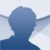# Mathway Android

4.0.2

## Mathway is a tool for Android devicescapable of solving complex math problems of all sorts, including statistics, algebra or trigonometry, amongst others

Mathway is much more than just a calculator for Android: it's a tool that solves math problems that range from basic calculations to complex chemistry-based problems.

### Solve operations and study all the steps taken

The greatest advantage of this application hasn't got to do with its capacity to solve different math problems by simply entering the different parameters, which in any case is also good stuff. Its main asset is that we can view the steps followed to solve the problem and reach the final result. Therefore, it's an excellent tool to study mathematics.

The interface brings together the typical calculator design with that of an instant messaging and chat application: we only have to enter the problem's data and the latter will be shown on the upper part of the screen, including all the steps to reach the solution, shown as a text message conversation.

The problems can be input manually or taking a photo of them, therefore, it couldn't be any easier. The app covers all the following math branches:

• Basic math.
• Pre-algebra.
• Algebra.
• Trigonometry.
• Pre-calculus.
• Calculus.
• Statistics.
• Finite math.
• Linear algebra.
• Chemistry.
• Graphics.

• Performance improvements.